College Physics : Gravitational Potential Energy

Example Questions

Example Question #21 : College Physics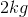bouncy ball is dropped from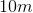. When it bounces back up is reaches a height of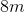. How much energy was loss?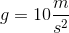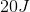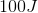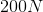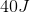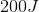Explanation:

The formula for gravitational potential energy is: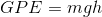To find the potential energy lost, we need to find the potential energy of the ball at two heights, then find the difference.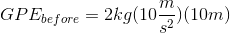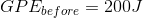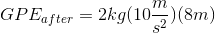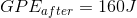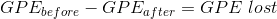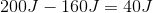Note that the energy was not actually lost; rather, it was converted to kinetic energy.

Example Question #22 : College Physics

A baseball weighingis dropped from a second story window which is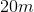high. What is the gravitational potential energy?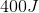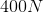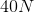Explanation:

Gravitational potential energy is given by the equation:We are given all the information needed to solve for the potential energy.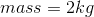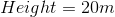Plug in known values and solve.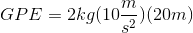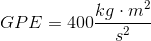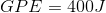Recall that the units for energy are Joules. Newtons is the unit for force.

Example Question #23 : College Physics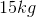bowling ball is released fromin the air. What is it's gravitation potential energy upon release?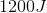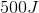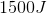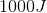Explanation:

The equation for gravitational potential energy is:We are given all the information needed to answer the question.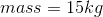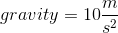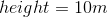Plug in known values and solve.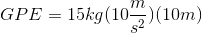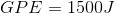All College Physics Resources